Thermodynamics

Chemical ThermodynamicsChemical thermodynamics is the study of the interrelation of heat and work with chemical reactions or with physical changes of state within the limits of the laws of thermodynamics.

Chemical thermodynamics involve not only laboratory measurements of various thermodynamic properties, but also the application of mathematical methods for the study of chemical questions and the spontaneity of processes.

The structure of chemical thermodynamics is based on the first two laws of thermodynamics. From the first law of thermodynamics and the second law of thermodynamics, four equations…

+ info

Laws Of Thermodynamics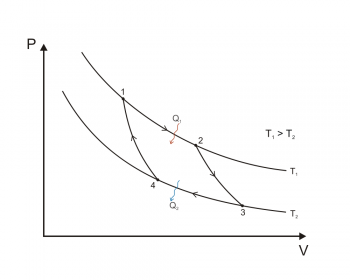Thermodynamics is mainly based on a set of four laws that are universally valid when applied to systems that fall within the constraints implicit in each.

The first principle that was established was the second law of thermodynamics, as formulated by Sadi Carnot in 1824. The 1860 already established two "principles" of thermodynamics with the works of Rudolf Clausius and William Thomson, Lord Kelvin. Over time, these principles have become "laws." In 1873, for example, Willard Gibbs claimed that there were two absolute laws of thermodynamics in his graphical methods in fluid thermodynamics.…

+ info

ThermodynamicsThermodynamics is the branch of classical physics that studies and describes the thermodynamic transformations induced by heat and work in a thermodynamic system, as a result of processes that involve changes in the temperature and energy state variables.

Classical thermodynamics is based on the concept of macroscopic system, that is, a portion of physical mass or conceptually separated from the external environment, which is often assumed for convenience that is not disturbed by the exchange of energy with the system. The state of a macroscopic system that is in equilibrium conditions…

+ info

Zero Law Of Thermodynamics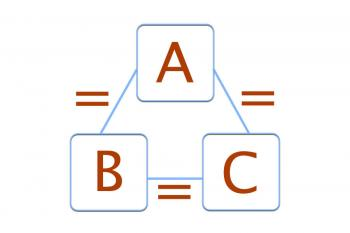The zero law of thermodynamics speaks of what we experience every day: two systems that are in thermal equilibrium with a third are in equilibrium with each other. It is said that two bodies are in thermal equilibrium when, on contacting each other, their state variables do not change. Around this simple idea the zero law is established.

Every law of physics has its relevance, as well as the zero law of thermodynamics, which curiously was the last law to be introduced in literature. After the realization that heat is a form of energy that could be transformed into another, thermology…

+ info

ThermodynamicsThermodynamics is the branch of physics that studies the effects of changes in temperature, pressure and volume of a physical system (a material, a liquid, a set of bodies, etc.), at a macroscopic level. The term "thermo" means heat and dynamics refers to motion, so thermodynamics studies the movement of heat in a body. Matter is composed of different particles that move disorderly. Thermodynamics studies this disorderly movement.

The practical importance of thermodynamics lies primarily in the diversity of physical phenomena it describes. Knowledge of this diversity has resulted in…

+ info

TemperatureDefinition of temperature: The temperature is the thermodynamic magnitude that shows the thermal energy of one body in relation to another.

Although the definition of temperature is simple and concise, you can explain what the temperature is in a more extensive way:

What is the temperature?

Temperature is a physical quantity of matter that quantifies the common notions of heat and cold. The objects of low temperature perceive them cold, while objects of higher temperatures we consider them warm or hot. This physiological sensation of cold and heat is generated…

+ info

Third Law Of Thermodynamics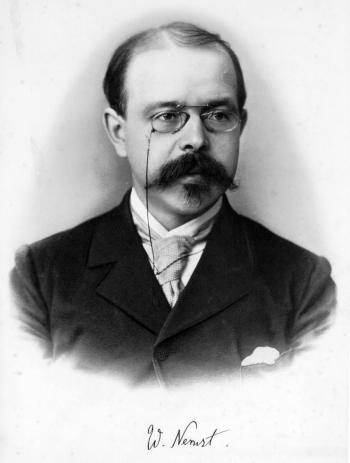The third law of thermodynamics, sometimes called Nernst's Theorem or Nernst's Postulate, relates the entropy and the temperature of a physical system.

The third law of thermodynamics states that absolute zero can not be achieved in a finite number of stages. The third law of thermodynamics can also be defined as that when reaching absolute zero, 0 degrees Kelvin, any process of a physical system stops and when reaching absolute zero the entropy reaches a minimum and constant value.

This principle states that the entropy of a system at the absolute zero temperature is a well-defined…

+ info

Second Law Of Thermodynamics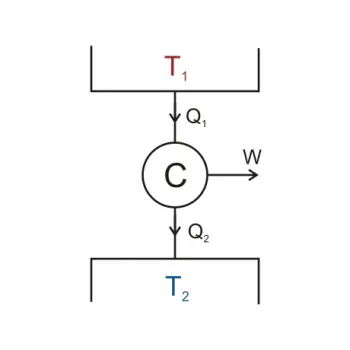According to the first law of thermodynamics, every process that occurs in a given system must satisfy the principle of conservation of energy, including the flow of heat.

Equation:

states, in other words, that any process whose sole purpose is to create or destroy energy, is impossible, that is, it denies the existence of a first-class perpetual motion machine.

However, the first law does not tell us anything about the…

+ info

First Law Of Thermodynamics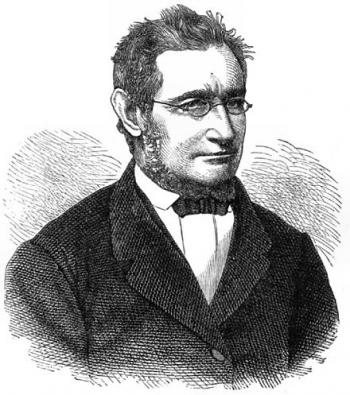The first law of thermodynamics was announced by Julius Robert von Mayer in 1841. It is the principle of conservation of energy.

Definition of the first law of thermodynamics: The total energy of an isolated system is neither created nor destroyed, it remains constant. Energy only transforms from one type to another. When one energy class disappears, an equivalent quantity of another class must be produced.

A body can have a certain speed with what has kinetic energy. If it loses speed, this kinetic energy that it loses becomes another type of energy, whether it is potential energy…

+ info

EntropyWhat is entropy? Entropy (S) is a thermodynamic quantity originally defined as a criterion for predicting the evolution of thermodynamic systems.

Entropy is a function of extensive character state. The value of entropy, in an isolated system, grows in the course of a process that occurs naturally. Entropy describes how a thermodynamic system is irreversible.

The meaning of entropy is evolution or transformation. The word entropy comes from the Greek.

Entropy in the world of physics

In physics, entropy is the thermodynamic magnitude that allows us to calculate the…

+ info

HeatIn physics, in particular in thermodynamics, heat is defined as the contribution of transformed energy as a result of a chemical or nuclear reaction and transferred between two systems or between two parts of the same system. This energy is not attributable to a job or a conversion between two different types of energy. Heat is, therefore, a form of transferred energy and not a form of energy contained as internal energy.

As the energy is exchanged, the heat is measured in the International System in joules. In practice, however, it is often still used as the unit of measurement of calories,…

+ info

Isothermal Process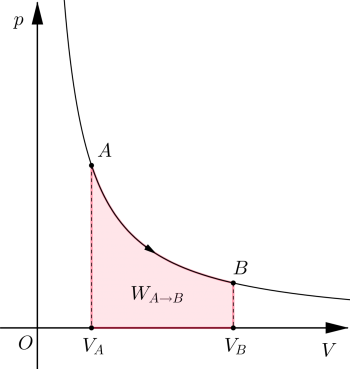In thermodynamics, an isothermal process is a thermodynamic transformation at constant temperature, that is, a variation of the state of a physical system during which the temperature of the system does not change with time. Devices called thermostats can maintain a constant temperature value.

The isothermal transformation of a perfect gas is described by Boyle's law which, in a pressure-volume diagram (or Clapeyron's plane), is represented by a branch of the equilateral hyperbola.

Isotherm of a perfect gas Calculation of heat and work exchanged

For isothermal gas…

+ info